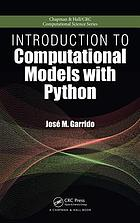Introduction to computational models with Python (eBook, 2016) [WorldCat.org]Preview this item
Checking...

# Introduction to computational models with Python

Author: José M Garrido Boca Raton, FL : CRC Press,  ©20 ©2016 Chapman & Hall/CRC computational science series.eBook : Document : EnglishView all editions and formats Introduction to Computational Models with Python explains how to implement computational models using the flexible and easy-to-use Python programming language. The book uses the Python programming language interpreter and several packages from the huge Python Library that improve the performance of numerical computing, such as the Numpy and Scipy modules. Divided ed into five sections, the book first introduces the basic models and techniques for designing and implementing problem solutions, independent of software and hardware tools. The second section discusses programming principles with the Python programming language, covering basic programming concepts, data definitions, programming structures with flowcharts and pseudo-code, solving problems, and algorithms. The third section describes Python lists, arrays, basic data structures, object orientation, linked lists and recursion, and running programs under Linux. In the fourth section, examples and case studies demonstrate the application of programming principles and fundamental techniques to relatively simple computational models. The final section focuses on the modeling of linear optimization problems, from problem formulation to implementation of computational models.  Read more... (not yet rated) 0 with reviews - Be the first.

## Find a copy in the libraryFinding libraries that hold this item...

## Details

Genre/Form: Electronic books Print version:Garrido, José M.Introduction to computational models with Python.Boca Raton, FL : CRC Press,  Document, Internet resource Internet Resource, Computer File José M Garrido Find more information about: José M Garrido 9781498712040 1498712045 923637941 "A Chapman & Hall book." 1 online resource (xxix, 466 pages) : illustrations Section 1. Problem solving -- section 2. Basic programming principles with Python -- section 3. Data structures, object orientation, and recursion -- section 4. Fundamental computational models with Python -- section 5. Linear optimization models. Chapman & Hall/CRC computational science series. José M. Garrido, Kennesaw State University, Kennesaw, Georgia, USA.

### Abstract:

Introduction to Computational Models with Python explains how to implement computational models using the flexible and easy-to-use Python programming language. The book uses the Python programming language interpreter and several packages from the huge Python Library that improve the performance of numerical computing, such as the Numpy and Scipy modules. Divided ed into five sections, the book first introduces the basic models and techniques for designing and implementing problem solutions, independent of software and hardware tools. The second section discusses programming principles with the Python programming language, covering basic programming concepts, data definitions, programming structures with flowcharts and pseudo-code, solving problems, and algorithms. The third section describes Python lists, arrays, basic data structures, object orientation, linked lists and recursion, and running programs under Linux. In the fourth section, examples and case studies demonstrate the application of programming principles and fundamental techniques to relatively simple computational models. The final section focuses on the modeling of linear optimization problems, from problem formulation to implementation of computational models.

## Reviews

User-contributed reviewsBe the first.

## Similar Items

### Related Subjects:(5)

Confirm this request

You may have already requested this item. Please select Ok if you would like to proceed with this request anyway.

Please sign in to WorldCat

Don't have an account? You can easily create a free account.